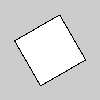Name

### rotateZ()

Examples```size(100, 100, P3D)
translate(width/2, height/2)
rotateZ(PI/3.0)
rect(-26, -26, 52, 52)
``````size(100, 100, P3D)
translate(width/2, height/2)
rect(-26, -26, 52, 52)
```
Description Rotates a shape around the z-axis the amount specified by the angle parameter. Angles should be specified in radians (values from 0 to PI*2) or converted to radians with the radians() function. Objects are always rotated around their relative position to the origin and positive numbers rotate objects in a counterclockwise direction. Transformations apply to everything that happens after and subsequent calls to the function accumulates the effect. For example, calling rotateZ(PI/2) and then rotateZ(PI/2) is the same as rotateZ(PI). If rotateZ() is called within the draw(), the transformation is reset when the loop begins again. This function requires using P3D as a third parameter to size() as shown in the examples above.
Syntax
```rotateZ(angle)
```
Parameters
angle float: angle of rotation specified in radians
Related popMatrix()
pushMatrix()
rotate()
rotateX()
rotateY()
scale()
translate()

Updated on Mon Sep 21 15:53:25 2020.

If you see any errors or have comments, please let us know.